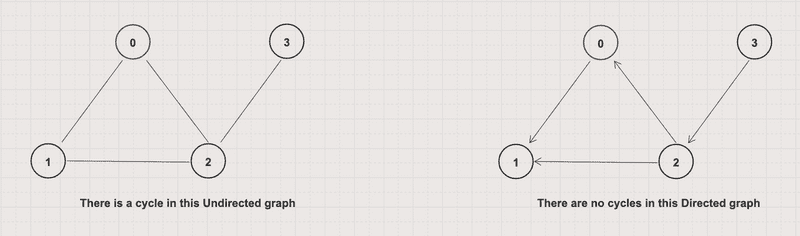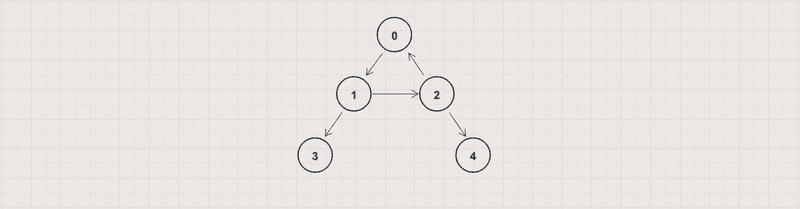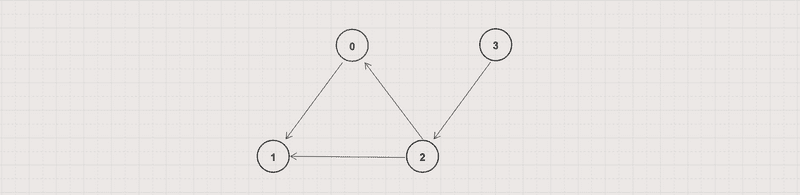# HelloKoding

Practical coding guides

# Detect Cycle in a Directed Graph

In this article, you will learn to use Depth-First Search algorithms to detect a cycle in a directed graph

Unlike in an undirected graph, to detect a cycle in a directed graph you should consider the direction of the edgesLearn about the type of graphs

Let’s get started!

## Problem

• Given a directed graph G = (V, E)
• Write an algorithm to detect a cycle in that graph

## Example 1

• Input: Given a directed graph G = (V, E) with V = 5 and E = [[0, 1], [1, 2], [2, 0], [1, 3], [2, 4]]• Output: true

## Example 2

• Input: Given a directed graph G = (V, E) with V = 4 and E = [[0, 1], [2, 0], [2, 1], [3, 2]]• Output: false

## Approach 1: DFS Iterative

• Use a stack to track the traversal path
• Keeping track of the visited and on-stack state of each vertex

While visiting a vertex and its adjacencies, if we ever encounter an on-stack vertex then the graph has a cycle

DetectCycleDirectedByDFSIterative.java

``````package com.hellokoding.datastructure.graph;

import java.util.ArrayDeque;
import java.util.Deque;

public class DetectCycleDirectedByDFSIterative {
boolean[] visited = new boolean[g.getV()];
boolean[] onStack = new boolean[g.getV()];
Deque<Integer> stack = new ArrayDeque<>();

for (int i = 0; i < g.getV(); i++) {
if (visited[i]) continue;
stack.push(i);

while (!stack.isEmpty()) {
int v = stack.peek();

if (!visited[v]) {
visited[v] = true;
onStack[v] = true;
} else {
onStack[v] = false;
stack.pop();
}

for (Integer w : g.getAdjacencyList().get(v)) {
if (!visited[w]) {
stack.push(w);
} else if (onStack[w]) {
return true;
}
}
}
}

return false;
}

public static void main(String[] args) {
System.out.println(dfsByIterative(g1));

System.out.println(dfsByIterative(g2));
}
}
``````

`GraphDirectedByAdjacencyList` is defined in Graph Data Structure Tutorial

• Output
``````true
false``````
• Time complexity: O(V+E)
• Space complexity: O(V)

## Approach 2: DFS Recursive

• Instead of using a stack, the algorithm calls to itself to explore unvisited vertices
• Keeping track of the visited and on-stack state of each vertex

While visiting a vertex and its adjacencies, if we ever encounter an on-stack vertex then the graph has a cycle

DetectCycleDirectedByDFSRecursive.java

``````package com.hellokoding.datastructure.graph;

import java.util.List;

public class DetectCycleDirectedByDFSRecursive {
static boolean dfsByRecursive(GraphDirectedByAdjacencyList g, int v, boolean[] visited, boolean[] onStack) {
if (onStack[v]) return true;
if (visited[v]) return false;

visited[v] = true;
onStack[v] = true;

for (Integer c: children) {
if (dfsByRecursive(g, c, visited, onStack)) {
return true;
}
}

onStack[v] = false;
return false;
}

boolean[] visited = new boolean[g.getV()];
boolean[] onStack = new boolean[g.getV()];

for (int i = 0; i < g.getV(); i++) {
if (dfsByRecursive(g, i, visited, onStack)) {
return true;
}
}

return false;
}

public static void main(String[] args) {
System.out.println(hasCycle(g1));

System.out.println(hasCycle(g2));
}
}
``````

`GraphDirectedByAdjacencyList` is defined in Graph Data Structure Tutorial

• Output
``````true
false``````
• Time complexity: O(V+E)
• Space complexity: O(V)

## Approach 3: DFS Iterative with Color

• Use a stack to track the traversal path
• Keeping track of the unvisited (White color), visit in progress (Gray color, the vertex is on the stack) and visited state (Black color) of each vertex

While visiting a vertex and its adjacencies, if we ever encounter a gray vertex then the graph has a cycle

DetectCycleDirectedByDFSIterativeColor.java

``````package com.hellokoding.datastructure.graph;

import java.util.ArrayDeque;
import java.util.Deque;

public class DetectCycleDirectedByDFSIterativeColor {
static final int WHITE = 0, GRAY = 1, BLACK = 2;

int[] color = new int[g.getV()];
Deque<Integer> stack = new ArrayDeque<>(g.getV());

for (int i = 0; i < g.getV(); i++) {
if (color[i] != WHITE) continue;
stack.push(i);

while (!stack.isEmpty()) {
int v = stack.peek();

if (color[v] == WHITE) {
color[v] = GRAY;
} else {
color[v] = BLACK;
stack.pop();
}

for (int w : g.getAdjacencyList().get(v)) {
if (color[w] == GRAY) {
return true;
} else if (color[w] == WHITE) {
stack.push(w);
}
}
}
}

return false;
}

public static void main(String[] args) {
System.out.println(dfsByIterativeWithColor(g1));

System.out.println(dfsByIterativeWithColor(g2));
}
}
``````

`GraphDirectedByAdjacencyList` is defined in Graph Data Structure Tutorial

• Output
``````true
false``````
• Time complexity: O(V+E)
• Space complexity: O(V)

## Approach 4: DFS Recursive with Color

• Instead of using a stack, the algorithm calls to itself to explore unvisited vertices
• Keeping track of the unvisited (White color), visit in progress (Gray color, the vertex is on the stack) and visited state (Black color) of each vertex

While visiting a vertex and its adjacencies, if we ever encounter a gray vertex then the graph has a cycle

DetectCycleDirectedByDFSRecursiveColor.java

``````package com.hellokoding.datastructure.graph;

public class DetectCycleDirectedByDFSRecursiveColor {
static final int WHITE = 0, GRAY = 1, BLACK = 2;

static boolean dfsByRecursiveWithColor(GraphDirectedByAdjacencyList g, int v, int[] color) {
color[v] = GRAY;

for (Integer w : g.getAdjacencyList().get(v)) {
if (color[w] == GRAY) {
return true;
}

if (color[w] == WHITE && dfsByRecursiveWithColor(g, w, color)) {
return true;
}
}

color[v] = BLACK;
return false;
}

int[] color = new int[g.getV()];

for (int i = 0; i < g.getV(); i++) {
if (color[i] == WHITE && dfsByRecursiveWithColor(g, i, color)) {
return true;
}
}

return false;
}

public static void main(String[] args) {
System.out.println(hasCycle(g1));

`GraphDirectedByAdjacencyList` is defined in Graph Data Structure Tutorial
``````true